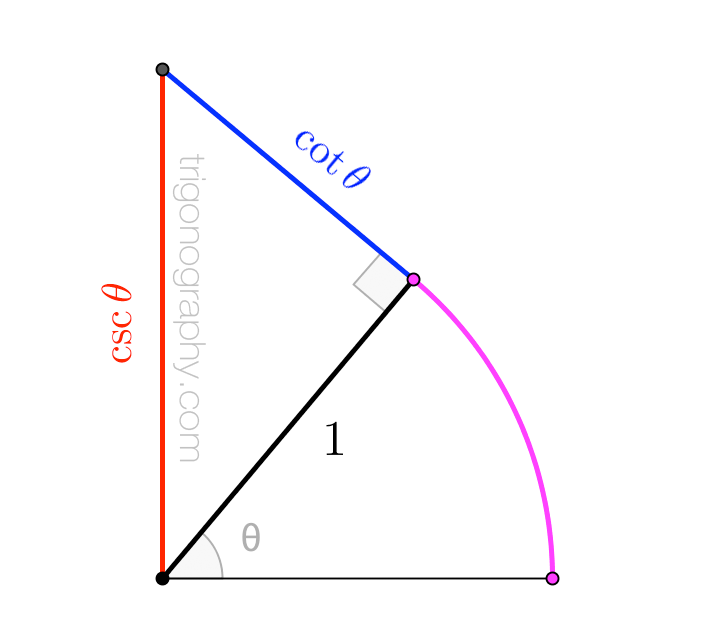## Trigonography Challenge: Series for Cotangent and Cosecant

Sine and cosine have their pinwheel. Secant and tangent have their zig-zag.

These raise an obvious question, to which I currently have no answer.
I offer it as the first-ever Trigonography Challenge:

What trigonograph depicts the (Laurent) series for cotangent and cosecant?\large \begin{align} \cot\theta &\;=\; \frac{1}{\theta} \;-\; \frac{1}{3}\theta \;-\; \frac{1}{45}\theta^3 \;-\; \frac{2}{945}\theta^5 \;-\; \cdots \\[6pt] &\;=\; \sum_{k=0}^{\infty} (-1)^k\,\frac{2^{2k}\,B_{2k}}{(2k)!}\;\theta^{2k-1} \\[12pt] \csc\theta &\;=\; \frac{1}{\theta} \;+\; \frac{1}{6}\theta \;+\; \frac{7}{360}\theta^3 \;+\; \frac{31}{15120} \theta^5 \;+\; \cdots \\[6pt] &\;=\; \sum_{k=0}^{\infty} (-1)^{k+1}\,\frac{\left(2^{2k}-2\right)B_{2k}}{(2k)!}\;\theta^{2k-1} \end{align}
where $$B_n$$ is the $$n$$-th (“first”) Bernoulli number.
A possible starting place is this “natural” construction of
the first term of the cotangent series, using an involute.Couple this with the fact that the remaining terms of the series are negative,
and then “all we need to do” is show that the length of the overhanging
sub-segment is given by the sum of (the absolute values of) those terms.

That, sadly, is about as far as I’ve gone with these series. Can you go farther?
If you can solve this Trigonography Challenge, please email or tweet.
There’s no prize, but I’ll post the best solution(s) here.